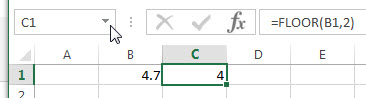# Excel Floor Function

This post will guide you how to use Excel FLOOR function with syntax and examples in Microsoft excel.

### Description

The Excel FLOOR function returns a number rounded down to the nearest multiple of significance. So it will return a rounded number.

The FLOOR function is a build-in function in Microsoft Excel and it is categorized as a Math and Trigonometry Function.
The FLOOR function is available in Excel 2016, Excel 2013, Excel 2010, Excel 2007, Excel 2003, Excel XP, Excel 2000, Excel 2011 for Mac.

### Syntax

The syntax of the FLOOR function is as below:

` = FLOOR (number, significance)`

Where the FLOOR function arguments are:

• Number -This is a required argument. The numeric value that you want to round.
• Significance – This is a required argument. The multiple to which you want to round

Note:

• If both above arguments is not numeric value, then the FLOOR function returns the #VALUE! Error value.
• If the number value is positive and the significance is negative, the FLOOR function returns the #NUM! Error value.
• If the significance argument is equal to 0, the FLOOR function returns the #DIV/0! Error.

### Excel FLOOR Function Example

The below examples will show you how to use Excel FLOOR function to round a supplied number to the nearest multiple of a specified significance.

#1 to round the value in B1 Cell down to nearest multiple of 2, just using the following excel formula:

`=FLOOR(B1,2)`